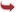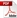International Journal of Scientific and Research Publications

#### IJSRP, Volume 6, Issue 5, May 2016 Edition [ISSN 2250-3153]Equations for Planetary Ellipses
Eric Sullivan
Abstract: Planetary orbits are ellipses with the sun at one of the foci. The semi major axis of each planetary orbital was used in part with each planets eccentricity to calculate the semi minor axis and the location of the foci. Equations in standard ellipse form were created for each of the planets. In the first model, the sun is placed at (0,0). With this set-up, the equations can be completely derived. Once the equations have been derived, the location of the sun was shifted to the positive (c,0) value. The distances for perihelion and aphelion were calculated by an adapted version of the standard distance equation. After this the accepted value for perihelion and aphelion for each planet was compared to the value measured. The percent error was then calculated.
[VIEW FULL PAPER][DOWNLOAD]

Reference this Research Paper (copy & paste below code):

Eric Sullivan (2018); Equations for Planetary Ellipses; Int J Sci Res Publ 6(5) (ISSN: 2250-3153). http://www.ijsrp.org/research-paper-0516.php?rp=P535356